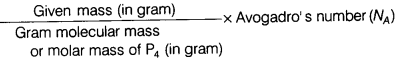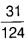# Calculate the number of particles

Calculate the number of particles in 31 g of P4 molecules. [Atomic mass of P = 31 u]

Molar mass of P4 molecules = 4 x atomic mass of P = 4 x 31 = 124 g
Number of particles (N) ==x 6.022 x 1023
N = 1.5 x 1023